Visual Case Based Type Questions: Chemical Reactions & Equations - 2

# Visual Case Based Type Questions: Chemical Reactions & Equations - 2 - Science Class 10

The following diagram displays a chemical reaction. Observe carefully and answer any questions: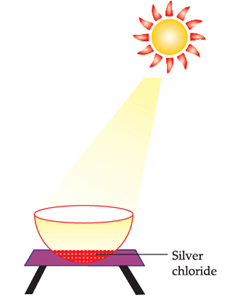Question 1:
The type of chemical reaction that will take place is
(a) Photochemical decomposition
(b) Displacement reaction
(c) Reduction reaction
(d) Combination reaction

Let’s look at all the options one-by-one
Photochemical decomposition: Photo means light, therefore the reactions in which a compound is broken down/decomposed in the presence of light are known as photochemical reactions.
Displacement reaction: Reaction in which more reactive element takes the place of less reactive element.
Reduction: The loss of oxygen is known as reduction.
Combination Reaction: When two or more substances react to form a single product, it is known as combination reaction.
Since the above reaction is taking place in the presence of light, it is a photochemical decomposition reaction.
So, the correct answer is (a)

Question 2:
When decomposition is carried out by heating, it is called as:
(a) Heat decomposition
(b) Photolytic decomposition
(c) Electrolytic decomposition
(d) Thermal decomposition

Thermal decomposition, or thermolysis, is a chemical decomposition caused by heat. The decomposition temperature of a substance is the temperature at which the substance chemically decomposes. The reaction is usually endothermic as heat is required to break chemical bonds in the compound undergoing decomposition.

Question 3:
The other silver salt which behaves like silver chloride in sunlight is:
(a) silver hydride
(b) silver bromide
(c) silver iodide
(d) silver nitrite

Silver Bromide behaves like Silver Chloride
So, the correct answer is (b)

Marble’s popularity began in ancient Rome and Greece, where white and off-white marble were used to construct a variety of structures, from handheld sculptures to massive pillars and buildings.
Question 4:
A student added 10g of calcium carbonate in a rigid container, secured it tightly and started to heat it. After some time, an increase in pressure was observed, the pressure reading was then noted at intervals of 5 minutes and plotted against time, in a graph as shown below. During which time interval did maximum decomposition took place?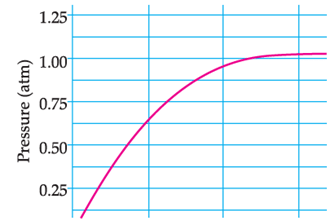(a) 15-20 min
(b) 10-15 min
(c) 5-10 min
(d) 0-5 min

This is the Pressure Time Graph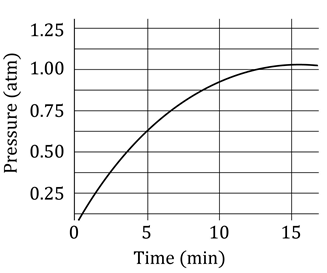Maximum decomposition is when the pressure is maximum
We see that from 0 to 5 minutes, the pressure increases from 0 to 0.625 atm, This indicates maximum increase in pressure due to maximum CO2 formation

Chemistry in Automobiles:
For an internal combustion engine to move a vehicle down the road, it must convert the energy stored in the fuel into mechanical energy to drive the wheels. In your car, the distributor and battery provide this starting energy by creating an electrical "spark", which helps in combustion of fuels like gasoline. Below is the reaction depicting complete combustion of gasoline in full supply of air:

2C8H18(l) + 25O2(g) → 16 'X' + Y
Question 5:
On the basis of evolution/absorption of energy, which of the following processes are similar to combustion of fuel?
(i) Photosynthesis in plants
(ii) Respiration in the human body
(iii) Decomposition of vegetable matter
(iv) Decomposition of ferrous sulphate.
(a) (ii) & (iii)
(b) (i) & (ii)
(c) (iii) & (iv)
(d) (ii) & (i)

In a Combustion reaction, heat is released (evolved)
Let’s check all the options:
In photosynthesis, the plants make food by absorbing the energy of the sun. So, this is not similar to Combustion
The breakdown of food to release energy is known as respiration. Hence, energy is released. So, this is similar to Combustion
Decomposition of vegetable matter involves release of energy. So, this is similar to Combustion
In order to carry out the decomposition of ferrous sulphate, we need to supply heat. So, this is not similar to Combustion
So, the correct answer is (a)

The physical states of the reactants and products can be represented by using the symbols (s) for solids, (l) for liquids, (g) for gases and (aq) for aqueous solution along with their respective formulae. The word aqueous is written if the reactant or product is present as a solution in water. Precipitate can also be represented by using an arrow pointing downwards (↓) instead of using symbol (s).
In the same way, the gaseous state of an evolved gas can be represented by using an arrow pointing upward direction (↑) instead of using symbol (g). The specific condition of the reaction like temperature, pressure, catalyst etc. is written above or below the arrow in the chemical equation.
Question 6:
The correct way to represent the evolution of gas, is to use which of the following symbol:
(a)
(b)
(c)
(d) (g)

Reaction of Magnesium with Hydrochloric Acid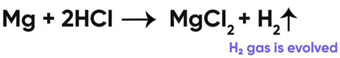So, the correct answer is (c) ↑

Question 7:
Which of the following reaction is balanced?
(a) NaCl + 2H2O → 2NaOH + 2Cl2 + H2
(b) 2NaCl + H2O → 2NaOH + 2Cl2 + H2
(c) 2NaCl + 2H2O → 2NaOH + Cl2 + H2
(d) 2NaCl + 2H2O → NaOH + Cl2 + H2

A balanced chemical equation is one in which the number of atoms of both reactants and products are equal.
In reaction I: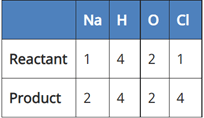Since the number of Na and Cl atoms in reactant and product is not equal, it is not a balanced equation.
In reaction II: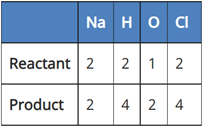Since the number of H, O and Cl atoms in reactant and product is not equal. It is not a balanced equation.
In reaction III: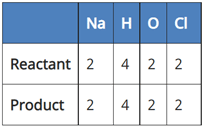Since the number of all atoms in reactants and products is equal, it is a balanced equation.
In Reaction IV: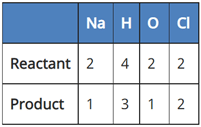Since the number of Na, H, and O atoms is not equal in reactant and product, it is not a balanced equation.
So, the correct answer is  (c) 2NaCl + 2H2O → 2NaOH + Cl2 + H2

In the following chemical reaction ‘‘zinc oxide reacts with carbon to produce zinc metal and carbon monoxide.’’ Answer any four question from I to V.
ZnO + C → Zn + CO
Question 8:
Name the type of reaction:
(a) oxidation reaction
(b) reduction reaction
(c) redox reaction
(d) decomposition reaction

Oxidation and Reduction Reaction Example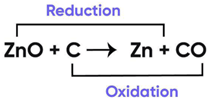Since both oxidation and reduction is taking place in this reaction, it is a redox reaction.
So, the correct answer is (c) redox reaction

Question 9:
Which of the following is the effect of oxidation reaction in everyday life:
(a) Precipitation
(b) Fermentation
(c) Corrosion
(d) Hydrogenation of oil

Let’s look at each of the reaction, one by one
Precipitation reaction reaction is not an oxidation reaction because this simply involves the formation of an insoluble substance (precipitate).
Fermentation breaking down of food in the absence of oxygen
Corrosion involves the oxidation of iron to form rust
Hydrogenation of oil is not an oxidation reaction because in this, hydrogen atoms are added over the double bonds of hydrocarbons.
So, the correct answer is (c) Corrosion

P, Q and R are 3 elements which undergo chemical reactions according to the following equations Answer any four question from I to V:
(i) P2O3 + 2Q → Q2O3 + 2P
(ii) 3RSO4 + 2Q → Q2(SO4)3 + 3R
(iii) 3RO + 2P → P2O3 + 3R

Question 10:
Choose the correct statement:

(a) Zinc and lead are more reactive elements than copper.
(b) Zinc and lead are less reactive elements than copper.
(c) Zinc and copper are more reactive elements than lead.
(d) Copper and lead are more reactive elements than zinc.

According to the reactivity series, Zinc and lead are more reactive than copper because they both are able to replace copper from its salt solution.

Question 11:
Na2SO4 (aq) + BaCl2(aq) → BaSO4 (s) + 2NaCl(aq)
The above reaction is an example of:
(a) Double displacement reaction.
(b) Displacement reaction.
(c) Can be both.
(d) None of the above.

Since in this reaction the two reacting compounds are switching their positive and negative ions, it is a double displacement reaction.
So, the correct answer is (a)

Question 12:
The type of reaction is:
(a) Displacement reaction
(b) Combination reaction
(c) Neutralisation reaction
(d) Substitution reaction

Let’s look at all the options
Displacement Reaction: Reaction in which more reactive element takes the place of less reactive element.
Combination Reaction: When two or more substances react to form a single product, it is known as combination reaction.
Neutralisation reaction: A reaction in which acid and base react to form salt and water
Substitution Reaction: In this reaction, one functional group in a chemical compound is replaced by another.
Since in the above mentioned reactions, the more reactive elements (Q, P) are replacing the less reactive element (R), this is a displacement reaction.
So, the correct answer is (a) Displacement reaction

The document Visual Case Based Type Questions: Chemical Reactions & Equations - 2 | Science Class 10 is a part of the Class 10 Course Science Class 10.
All you need of Class 10 at this link: Class 10

## Science Class 10

78 videos|509 docs|153 tests

## FAQs on Visual Case Based Type Questions: Chemical Reactions & Equations - 2 - Science Class 10

 1. What is a chemical reaction?A chemical reaction is a process in which one or more substances, known as reactants, interact to form different substances, known as products. During a chemical reaction, the bonds between atoms in the reactants are broken and new bonds are formed to create the products.
 2. How are chemical reactions represented?Chemical reactions are represented using chemical equations. In a chemical equation, the reactants are written on the left side and the products are written on the right side, separated by an arrow. The number of each type of atom is balanced on both sides of the equation to satisfy the law of conservation of mass.
 3. What is a balanced chemical equation?A balanced chemical equation is an equation in which the number of each type of atom is the same on both sides. This means that the mass is conserved during the reaction. To balance an equation, coefficients are added in front of the reactants and products to adjust the number of atoms.
 4. How can we determine the type of chemical reaction?The type of chemical reaction can be determined based on the changes in the chemical properties and composition of the substances involved. Some common types of chemical reactions include synthesis (combination), decomposition, combustion, displacement, and double displacement reactions.
 5. Why is it important to balance chemical equations?Balancing chemical equations is important because it ensures that the law of conservation of mass is satisfied. This law states that matter cannot be created or destroyed during a chemical reaction, only rearranged. By balancing the equation, we can accurately determine the quantities of reactants and products involved in the reaction.

## Science Class 10

78 videos|509 docs|153 testsExplore Courses for Class 10 examSignup to see your scores go up within 7 days! Learn & Practice with 1000+ FREE Notes, Videos & Tests.
10M+ students study on EduRev
Track your progress, build streaks, highlight & save important lessons and more!
Related Searches

,

,

,

,

,

,

,

,

,

,

,

,

,

,

,

,

,

,

,

,

,

;